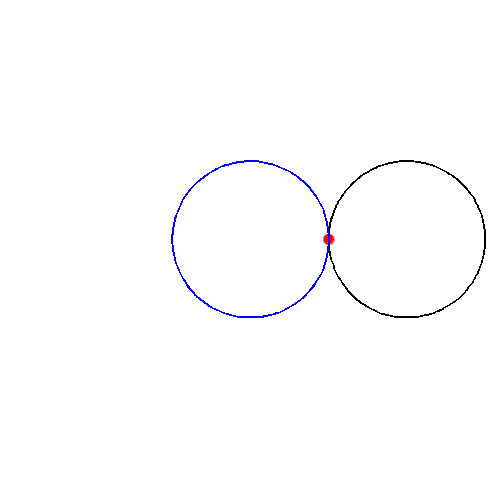UNCATEGORIZED

# Cardioid

[latexpage]

In my multivariable calculus class, I learned about various types of curves that take different formats, from parametric to polar coordinates. Because the course was a sort of introduction to these notions, we weren’t given the “full” explanation on a bunch of these curves. Therefore, I want to touch on some interesting aspects of this category of curves (called limaçons) below.

First, I was only introduced to the curve by having the formula given to me. The formula was in polar coordinates, and is as follows:

$r=a(1+cos(\theta))$

As one can imagine, this formula makes little sense to a student when they haven’t learned much about these types of curves. However, what if they looked at this instead?By AtomicShoelace

When I first saw this, I just thought, “Oh, that’s what it is.” The curve makes so much more sense when viewed in this type of frame. I am confused as to why this animation wasn’t shown to my class, because we saw a similar animation for the cycloid.

What’s cool about the cardioid is that it’s a curve that has a very nice formula to compute its arc length. The formula is $L=16a$.

In terms of the area of a cardioid, it’s formula is pretty nice, too: $A=6\pi a^2$, which means it’s six times the area of the circles above. I always find it neat to see comparisons of different shapes to see how they relate to one another.More to come on these kinds of curves and ways to represent them.

Reference: Wikipedia